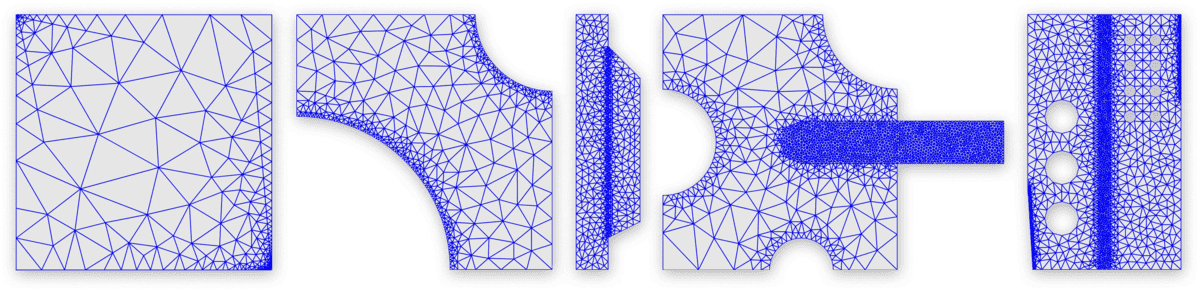# Calling the External Grid Generator Triangle from FEAToolPlease Note that the gridgen_triangle function has been updated and is now fully integrated with both the FEATool GUI and CLI. See the FEATool-Triangle Mesh Generator Integration post for more details.

FEATool Multiphysics supports calling external programs for grid generation, solvers, and postprocessing directly from the GUI. This post illustrates how this can be done by showing an example with Triangle, a very fast 2D unstructured grid generation code written by Jonathan R. Shewchuk.

1. The first step is to download and compile Triangle from www.cs.cmu.edu/~quake/triangle.html. Alternatively, precompiled mex files can found in the mutils package, and a Windows executable is also available from Sourceforge.

2. To use Triangle with FEATool put the executable binary in the featool/lib/triangle directory (create the directory if it doesn’t exist).

3. Also download the gridgen_triangle.m function wrapper from the link at the bottom of this post and put it in the featool directory (where also the gridgen function resides).

4. To use an alternative grid generation routine in the FEATool GUI, simply choose the Grid Generation Call… from the Grid menu.

5. This will open a dialog box where you can specify the grid generation call. In this case simply replace gridgen with the wrapper gridgen_triangle. Click on Generate Grid to call the specified grid generation routine.

Although DistMesh, the built-in automatic grid generation routine, is very robust and produces high quality grids. As can be seen from the table below Triangle can be a magnitude or more faster.

n_cellst (DistMesh)n_cellst (Triangle)
2110.401680.08
8990.356940.06
36421.1027240.08
146322.88108640.20
5869813.29438980.48
23579360.381709961.74

It also possible to call the Triangle grid generation function directly on the command line and directly import the created grid struct in to the GUI. The example script below generates a grid for a square domain with a circle subtracted from the upper right corner and compares it with DistMesh

``````hmax = 0.02;

xmin  = 0;
xmax  = 1;
ymin  = 0;
ymax  = 1;
gobj1 = gobj_rectangle( xmin, xmax, ymin, ymax, 'R1' );

xc    = 0.8;
yc    = 0.8;
r     = 0.4;
gobj2 = gobj_circle( [xc yc], r, 'C1' );

fea.geom.objects = { gobj1 gobj2 };
fea = geom_apply_formula( fea, 'R1-C1' );

tic
grid1 = gridgen( fea, 'hmax', hmax );
t_distmesh = toc;

tic
grid2 = gridgen_triangle( fea, 'hmax', hmax );
t_triangle = toc;

subplot(1,2,1)
plotgrid( grid1 )
title('DistMesh')
subplot(1,2,2)
plotgrid( grid2 )
title('Triangle')

t_distmesh
t_triangle
``````

The Triangle function wrapper also supports specifying the minimum acceptable angle and target grid sizes for each edge, for more information type `help gridgen_triangle` to see the help documentation. The function wrapper can be downloaded from the link below

FEATool-Triangle Mesh Generator Integration

Category: grid

Tags: external triangle